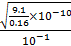# In an experiment electrons are accelerated, from rest, by applying a voltage of 500 V. calculate the radius of the path if a magnetic field 100 mT is then applied. [charge of the electron=1.6×10−19C Mass of the electron =9.1×10−31kg] (a) 7.5×10−4m (b) 7.5×10−3 m (c) 7.5 m (d) 7.5×10−2 m

## Question ID - 50306 :- In an experiment electrons are accelerated, from rest, by applying a voltage of 500 V. calculate the radius of the path if a magnetic field 100 mT is then applied. [charge of the electron=1.6×10−19C Mass of the electron =9.1×10−31kg] (a) 7.5×10−4m (b) 7.5×10−3 m (c) 7.5 m (d) 7.5×10−2 m

3537

r=r==r==×10−4=7.5×10−4

Next Question :
 A particle undergoing simple harmonic motion has time dependent displacement given by x(t)=Asin. The ratio of kinetic to potential energy of this particle at t=210 s will be: